# Ust

### Ust Oldfield's Blog

Forecasting is pretty crucial to any business as nearly every function within a company needs an estimate of what the future will look like as a foundation to create and update plans. In a previous blog I addressed the principles and methods of forecasting and in this blog I will go through how you can forecast in R and SQL using the Autoregressive Integrated Moving Average (ARIMA) technique.

I will approach this in two parts. Part 1 will be demonstrating the forecast in an R platform; and in Part 2 I will be demonstrating the forecast using the R integration in SQL Server in conjunction with SSRS to visualise the results.

Part 1: The Pure R Approach

As mentioned, this will be creating a forecast using R and taking data from a SQL database. You will need to be able to connect SQL to R in order to do follow this section. You’ll also need to install the forecast CRAN package. As always we’ll be using AdventureWorks for the provision of data.

The R script is as follows:

library(RODBC)

library(forecast)

SQLConnection <- odbcConnect("AdventureWorks")

Data <- sqlQuery(SQLConnection, "WITH CTE AS(SELECT YearDate as[Year], , , , , , , , , , , ,   FROM (

SELECT * FROM (SELECT --DISTINCT

SUM(salesamount) as monthsales

,MONTH([OrderDate]) as monthdate

,YEAR(OrderDate) as  yeardate

FROM [AdventureworksDW2016CTP3].[dbo].[FactInternetSales]

group by MONTH(orderdate), year(orderdate)) t )

AS Table1

PIVOT(SUM(MonthSales) FOR Monthdate IN(,,,,,,,,,,,)) AS PVT

)

SELECT [Year], cast( as numeric(28,8)) as Jan, cast( as numeric(28,8)) as Feb, cast( as numeric(28,8)) as Mar, cast( as numeric(28,8)) as Apr, cast( as numeric(28,8)) as May,

cast( as numeric(28,8)) as Jun, cast( as numeric(28,8)) as Jul, cast( as numeric(28,8)) as Aug, cast( as numeric(28,8)) as Sep, cast( as numeric(28,8)) as Oct,

cast( as numeric(28,8)) as Nov, cast( as numeric(28,8)) as [Dec] FROM CTE

ORDER BY [Year] ASC")

month <- c(1,2,3,4,5,6,7,8,9,10,11,12)

mn<- month.abb[month]

DataMonth <- Data[ ,mn]

DataTS<- ts(c(t(DataMonth)),start = c(2010,12), end = c(2014,1),frequency=12)

ForecastArima <- forecast(auto.arima(DataTS))

plot(ForecastArima)

ForecastArima

The numerical output will look like this: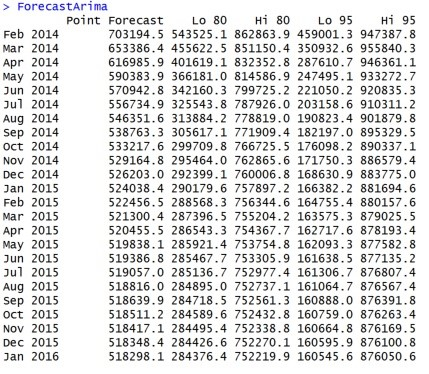With the graphical output should look something like this: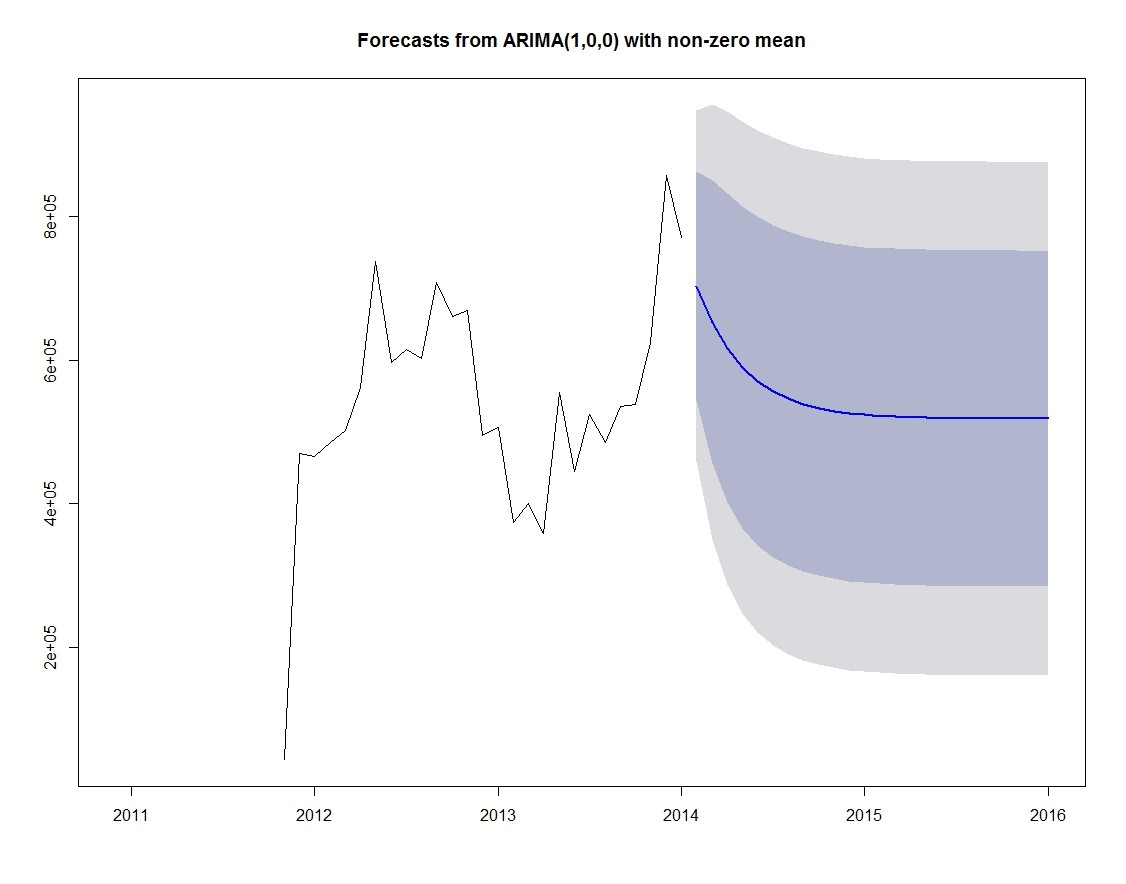Part 2: Forecasting in SQL

Forecasting in SQL follows a pretty similar approach to the pure R approach in producing the numerical output. But that’s where the similarity ends. As we’ll want to visualise the output we’ll need to do some manipulation on the result from the R script we will have to wrap the initial query in a stored procedure which can be called by the stored procedure which will populate the graph in SSRS.

The first step is to write the initial stored procedure which will contain the R script

CREATE PROCEDURE dbo.spForecastSalesPreparation AS

BEGIN

EXECUTE sp_execute_external_script

@language = N'R'

,@script = N'library(forecast);

Data<- InputDataSet

month<- c(1,2,3,4,5,6,7,8,9,10,11,12)

mn<-month.abb[month]

DataMonth <- Data[ ,mn]

DataTS <- ts(c(t(DataMonth)), start = c(2010,12), end = c(2014,1), frequency =12)

ForecastArima <- forecast(auto.arima(DataTS))

ForecastArimadf <- data.frame(ForecastArima)'

,@input_data_1 = N'WITH CTE AS(SELECT YearDate as[Year],,,,,,,,,,,,  FROM (

SELECT * FROM (SELECT

SUM(salesamount) as monthsales

,MONTH([OrderDate]) as monthdate

,YEAR(OrderDate) as  yeardate

FROM [AdventureworksDW2016CTP3].[dbo].[FactInternetSales]

group by MONTH(orderdate), year(orderdate)) t )

AS Table1

PIVOT(SUM(MonthSales) FOR Monthdate IN(,,,,,,,,,,,)) AS PVT)

SELECT [Year],

cast( as numeric(28,8)) as Feb,

cast( as numeric(28,8)) as Mar,

cast( as numeric(28,8)) as Jan,

cast( as numeric(28,8)) as Apr,

cast( as numeric(28,8)) as May,

cast( as numeric(28,8)) as Jun,

cast( as numeric(28,8)) as Jul,

cast( as numeric(28,8)) as Aug,

cast( as numeric(28,8)) as Sep,

cast( as numeric(28,8)) as Oct,

cast( as numeric(28,8)) as Nov,

cast( as numeric(28,8)) as [Dec] FROM CTE

ORDER BY [Year] ASC'

,@output_data_1_name = N'ForecastArimadf'

WITH RESULT SETS (("Point.Forecast" numeric(28,2) NOT NULL,

"Lo.80" NUMERIC(28,2) NOT NULL,

"Hi.80" NUMERIC(28,2) NOT NULL,

"Lo.95" NUMERIC(28,2) NOT NULL,

"Hi.95" NUMERIC(28,2) NOT NULL));

END;

The R script that you invoke is very similar to the one in the pure R approach, as is the SQL query. But the key element is the WITH RESULT SETS command, which returns the results of the query in a tabular format. Note, it is important to specify the data types for each of the columns you wish to return.

Because of the desire to visualise the results from R we’ll need to join the output from R with the input to R and keep it in a structure that makes sense, both as a table and as a graph. The stored procedure that I’ve created is as follows:

CREATE PROCEDURE dbo.spForecastSales AS

BEGIN

DECLARE @ForecastResults TABLE (Id INT IDENTITY(1,1),

Value NUMERIC(28,2),

Lo80 NUMERIC(28,2),

Hi80 NUMERIC(28,2),

Lo95 NUMERIC(28,2),

Hi95 NUMERIC(28,2))

DECLARE @FinalResults TABLE (Id INT IDENTITY(1,1),

ResultType VARCHAR(10),

YearDate INT,

MonthDate INT,

Value NUMERIC(28,2),

Lo80 NUMERIC(28,2),

Hi80 NUMERIC(28,2),

Lo95 NUMERIC(28,2),

Hi95 NUMERIC(28,2))

-- insert the actual sales values

INSERT INTO @FinalResults (ResultType,

YearDate,

MonthDate,

Value)

SELECT       'Actual'

,YEAR(OrderDate)   as  YearDate

,MONTH(OrderDate)  as      MonthDate

,SUM(SalesAmount)  as    Value

FROM [dbo].[FactInternetSales]

GROUP BY MONTH(OrderDate), YEAR(OrderDate)

ORDER BY YearDate ASC, MonthDate ASC

-- insert the forecast sales values

INSERT INTO @ForecastResults

EXECUTE dbo.spForecastSalesPreparation

DECLARE @MaxId INT,

@LastDate DATE;

SET @MaxId = (SELECT MAX(Id) FROM @FinalResults)

SELECT @LastDate = DATEFROMPARTS(YearDate, MonthDate, 1)

FROM @FinalResults

WHERE Id = @MaxId

INSERT INTO @FinalResults (ResultType,

YearDate,

MonthDate,

Value,

Lo80,

Hi80,

Lo95,

Hi95)

SELECT 'Forecast',

YearDate = DATEPART(year, DATEADD(month, Id, @LastDate)),

MonthDate = DATEPART(Month, DATEADD(month, Id, @LastDate)),

Value,

Lo80,

Hi80,

Lo95,

Hi95

FROM @ForecastResults

ORDER BY Id ASC

SELECT  Id,

ResultType,

DATEFROMPARTs(YearDate, MonthDate, 1) AS YearDate2,

Value,

Lo80,

Hi80,

Lo95,

Hi95

FROM @FinalResults

END;

Following the normal steps of creating a report in SSRS using a stored procedure as a dataset your graph might look something like this: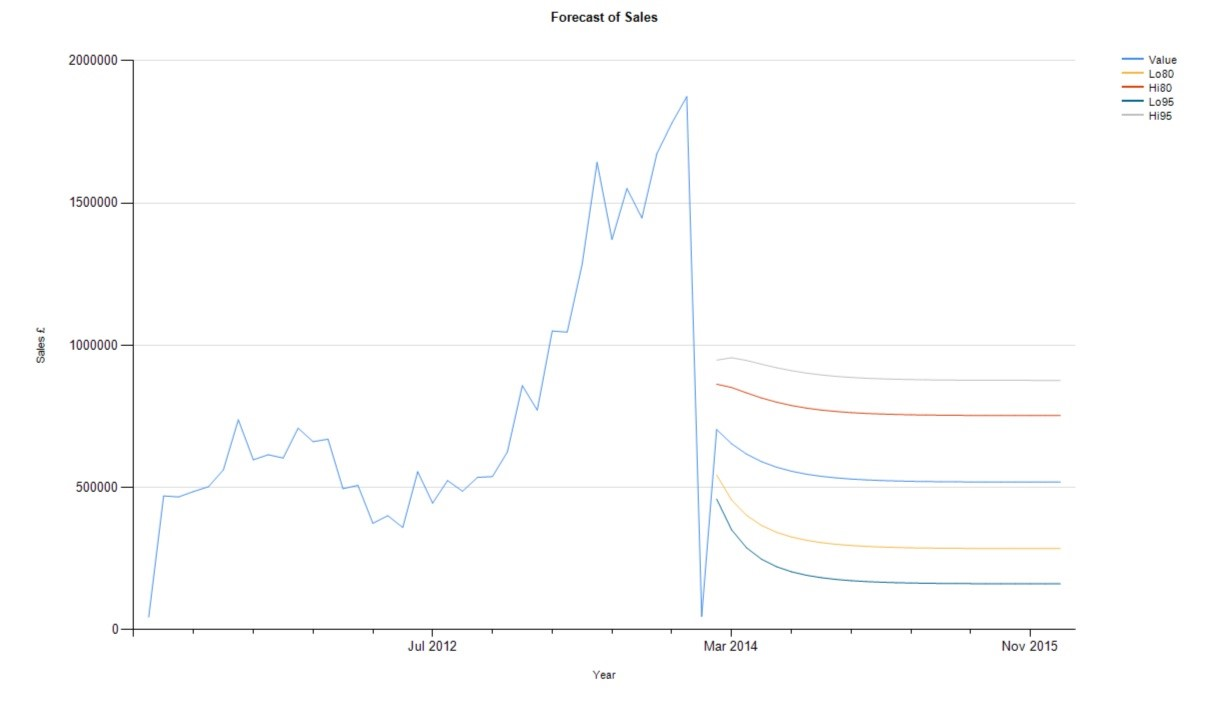So, we’ve shown two similar yet different approaches to forecasting using the ARIMA method and utilising R and SQL. Hopefully this demonstrates how quick and easy it is to establish a rudimentary forecasting practice wherever you are.

With the release of SQL Server 2016 CTP3 the inclusion of R has been a powerful and welcome addition. As R can now be directly queried from inside SQL Server Management Studio (SSMS) one can now use the data outputs from R in SQL Server Reporting Services (SSRS) thereby utilising the power of R in the convenience of SSRS.

This blog will guide you through the process of creating a simple report in SSRS using data from R.

As demonstrated in a previous blog, it is very easy to begin using R within SQL Server and this is no different.

First of you will need your SQL R Script, for which I’m producing a simple K Means cluster of employees in the Adventure Work Data Warehouse. Then you will want to wrap that query inside a stored procedure.

CREATE PROCEDURE dbo.spKMeansEmployee

AS

BEGIN

EXECUTE sp_execute_external_script

@language = N'R',

@script = N'ClusterCount <- 4;

df <- data.frame(InputDataSet);

ClusterFeatures <- data.frame(df\$BaseRate, df\$VacationHours, df\$SickLeaveHours, df\$SalaryFlag);

ClusterResult <- kmeans(ClusterFeatures, centers = ClusterCount, iter.max = 10)\$cluster;

OutputDataSet <- data.frame(df, ClusterResult);',

@input_data_1 = N'SELECT

EmployeeKey,

BaseRate,

VacationHours,

SickLeaveHours,

CAST(SalariedFlag AS VARCHAR(1)) AS SalaryFlag

FROM dbo.DimEmployee;'

WITH RESULT SETS (( EmployeeKey INT NOT NULL,

BaseRate MONEY NOT NULL,

VacationHours INT NOT NULL,

SickLeaveHours INT NOT NULL,

SalaryFlag VARCHAR(1) NOT NULL,

ClusterResult INT NOT NULL

));

END

The next step is to create a new report in Visual Studio and add a new Data Source.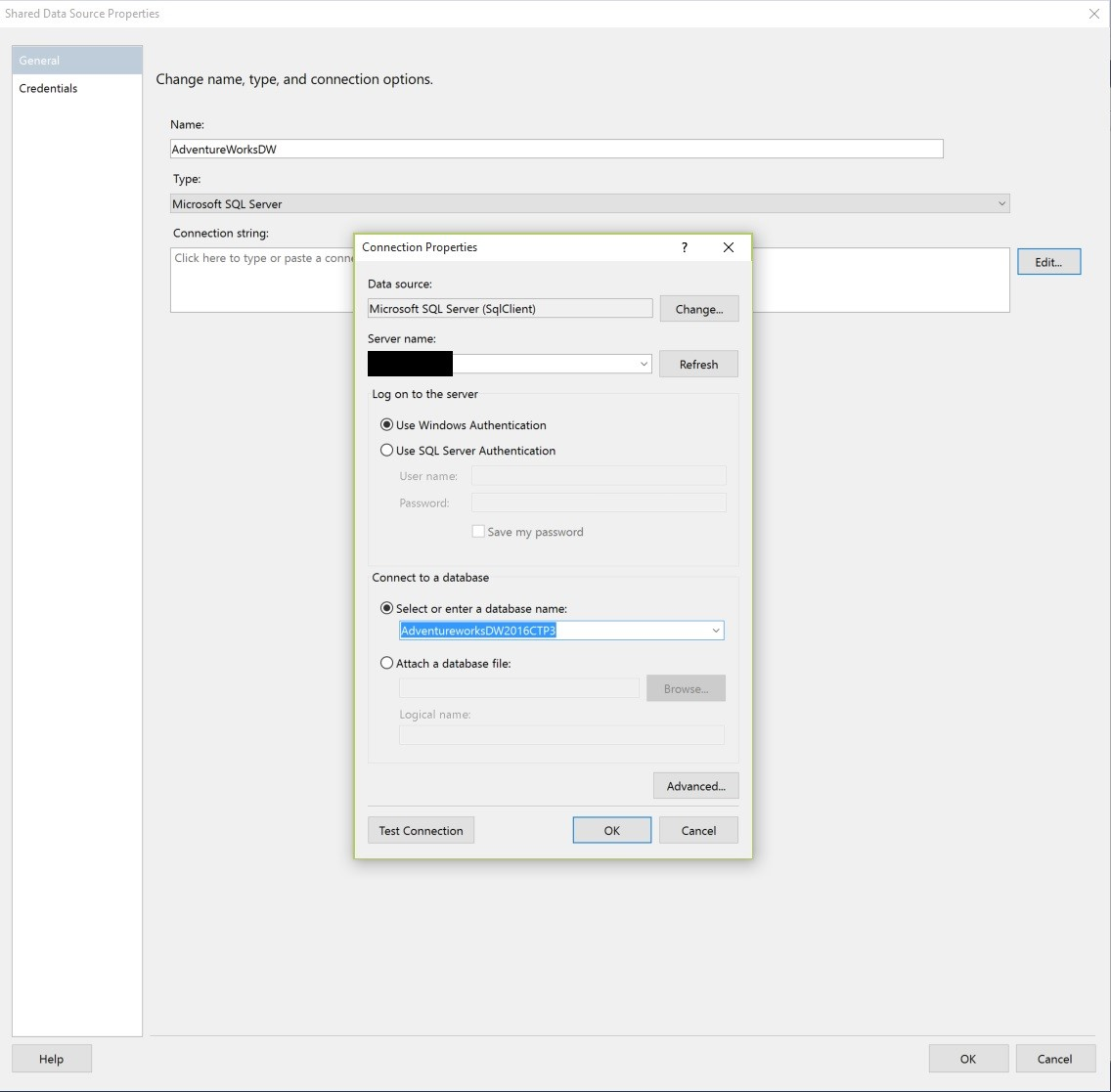Then create a dataset.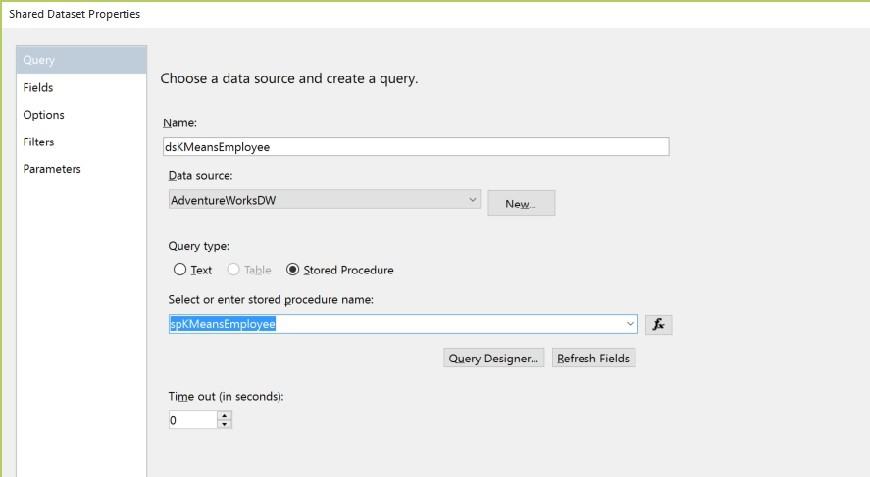And link that dataset to a new report.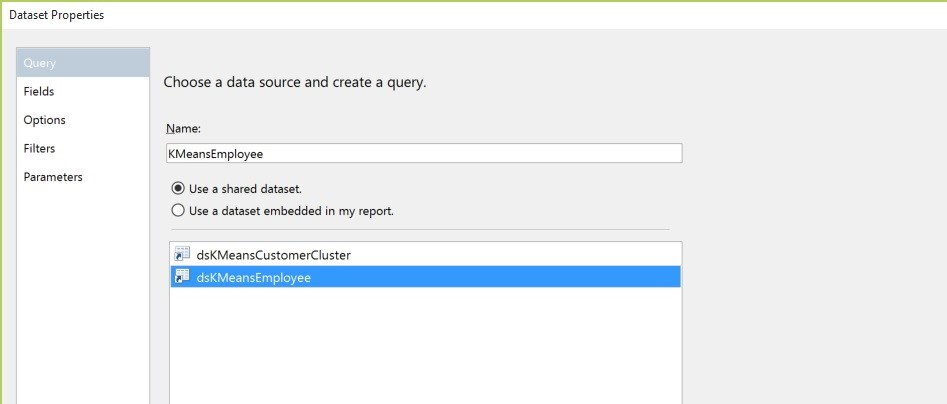Then build the report how you want, using that dataset. This is the quick output I’ve opted for as you can quickly analyse employees based on the cluster they are in.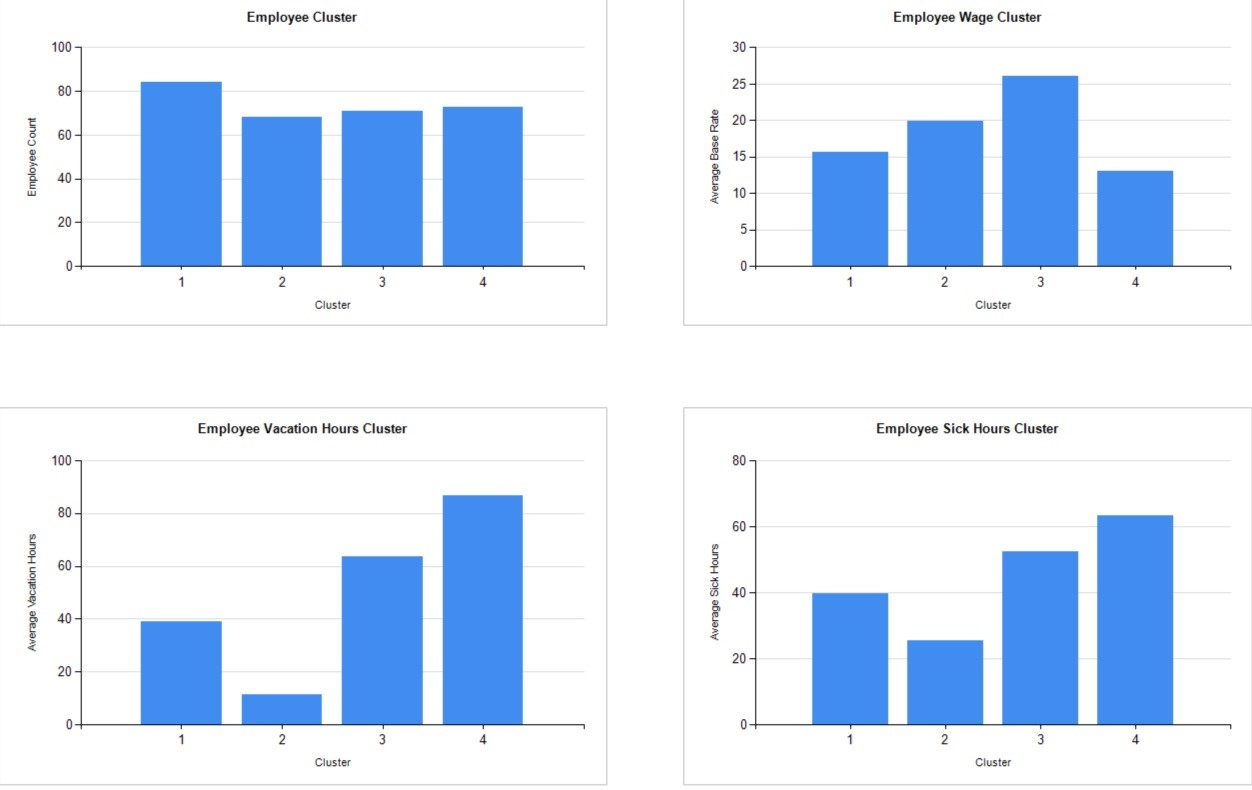As you can see, and hopefully reproduce, it’s a very quick and relatively easy process that allows you to make business decisions by utilising the combined powerful capabilities of R and SQL.

The release of SQL Server 2016 CTP3 is pretty big as it’s the first CTP which incorporates in-database support for R. This means that you can call R scripts and functions within a SQL query without any workarounds as previously demonstrated.

To get yourself up and running on CTP3 you’ll need to follow these steps:

### 1)      Download, install and configure CTP3 https://www.microsoft.com/en-us/evalcenter/evaluate-sql-server-2016

a.       Make sure that Advanced Analytics Extension is installed

b.       verify that the service for Advanced Analytics Extensions starts automatically using the MSSQLLaunchpad account.

c.        On the Database Engine Configuration page, for Authentication mode, select Mixed Mode (SQL Server authentication and Windows authentication).

i.      For CTP3, a SQL login is required when using the RevoScaleR functions from a remote workstation to run R scripts in the context of the server.

### 4)      Enable external scripts

exec sp_configure 'external scripts enabled',1;

RECONFIGURE;

### 5)      Run the post-installation script(s)

a.       Open an instance of command prompt with administrator privileges and run the following script

b.       C:\Program Files\RRO\RRO-3.2.2-for-RRE-7.5.0\R-3.2.2\library\RevoScaleR\rxLibs\x64\RegisterRExt.exe /install

i.      More info can be found here: https://msdn.microsoft.com/en-us/library/mt590536.aspx

Now you should be up and running and ready to fire up a new instance of SQL Server Management Studio to begin working with R. For the rest of the demo I will be using the latest version of Adventure Works for SQL 2016 CTP3, which can be found here: https://www.microsoft.com/en-us/download/confirmation.aspx?id=49502 Please restore the backed up databases to continue.

To start off we’re just going to do something really simple, which is return the mean of the UnitPrice from Sales.SalesOrderDetail in a result set, just to demonstrate functionality and ease in which you can write and execute R scripts within SQL.

The script I’m going to use is:

USE AdventureWorks2016CTP3;

EXECUTE sp_execute_external_script

@language = N'R'

,@script = N'OutputDataSet <-data.frame(mean(InputDataSet[,1]))'

,@input_data_1 = N'SELECT UnitPrice FROM [Sales].[SalesOrderDetail]'

WITH RESULT SETS ((col int not null));

What it does is call a stored procedure with three input parameters: @language; @script; and @input_data_1

The only valid input for @language is 'R’.

@script is the external language script specified as a literal or variable input. Datatype of @script is NVARCHAR(MAX).

@input_data_1 specifies the input data for the external script in the form of a T-SQL query. Datatype of @input_data_1 is NVARCHAR(MAX).

With the specification of _1 it would appear that in future one might be able to pass in more than one lot of data.

The syntax for sp_execute_external_script can be much more complex than demonstrated and more information can be found here: https://msdn.microsoft.com/en-us/library/mt604368.aspx

So, we’ve run our script. SQL has initiated the R script, which is taking a mean of the first column and converting it to a data frame. A data frame is similar to a table and, at the moment, the output data has to be in a data frame structure in order for it to be passed back into SQL. The end result is that the mean of the UnitPrice has been returned.

Query and result should look something like this:

R is a really powerful tool as it is and by incorporating in-database support for R within SQL Server 2016 allows for a convenient, usable and even more powerful toolkit to process and analyse data. The possibilities of application to your data are vast but can range from simple data exploration, as demonstrated above, to predictive modelling.

Be on the lookout for more demonstrations of R functionality within SQL Server 2016.